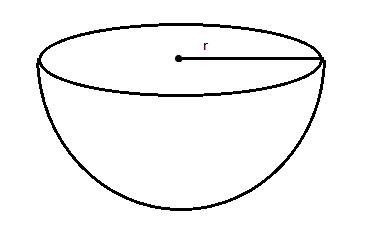QuestionAnswers

# The surface area of a solid hemisphere with radius $r$isA. $4\pi {r^2}$B. $2\pi {r^2}$C. $3\pi {r^2}$D. $\dfrac{2}{3}\pi {r^2}$

Hint: The total surface area of a solid hemisphere $\left( S \right)$with radius $r$ is equal to the sum of the curved surface area of the solid hemisphere and the flat surface area of the hemisphere. So, use this concept to reach the solution of the given problem.

Complete step-by-step solution -
The surface area of solid sphere with radius $r$= $4\pi {r^2}$
The diagram of solid hemisphere is shown as below:A solid sphere can be divided into two equal hemispheres with a flat surface and a curved surface.
Curved surface area of a solid hemisphere will be half the surface area of solid hemisphere $= \dfrac{1}{2} \times 4\pi {r^2} = 2\pi {r^2}$
And, the flat surface area of the hemisphere is equal to the area of the circle with radius $r$.
Hence, flat surface area of hemisphere = $\pi {r^2}$. Since the area of circle with radius $r$is $\pi {r^2}$
Therefore, the total surface area of a solid hemisphere $\left( S \right)$with radius $r$ is equal to the sum of the curved surface area of the solid hemisphere and the flat surface area of the hemisphere.
$\Rightarrow S = 2\pi {r^2} + \pi {r^2} \\ \therefore S = 3\pi {r^2} \\$
Thus, the correct option is C. $3\pi {r^2}$

Note: The surface area of the solid sphere with radius $r$= $4\pi {r^2}$. A solid sphere can be divided into two equal hemispheres with a flat surface and a curved surface. The flat surface area of the hemisphere is equal to the area of the circle with radius $r$.